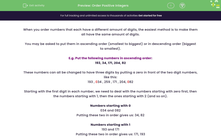# Order Positive Integers

In this worksheet, students will practise ordering positive numbers using place value into ascending or descending order, plus locating which numbers are in specific positions within these sequences.Key stage:  KS 4

Year:  GCSE

GCSE Subjects:   Maths

GCSE Boards:   Pearson Edexcel, OCR, Eduqas, AQA,

Curriculum topic:   Number, Algebra

Curriculum subtopic:   Structure and Calculation Algebraic Inequalities

Popular topics:   Numbers worksheets, Place Value worksheets

Difficulty level:#### Worksheet Overview

When you order numbers that each have a different amount of digits, the easiest method is to make them all have the same amount of digits.

You may be asked to put them in ascending order (smallest to biggest) or in descending order (biggest to smallest).

E.g. Put the following numbers in ascending order:

193, 34, 171, 204, 82

These numbers can all be changed to have three digits by putting a zero in front of the two digit numbers, like this:

193 , 034 , 259 , 171 , 204, 082

Starting with the first digit in each number, we need to deal with the numbers starting with zero first, then the numbers starting with 1, then the ones starting with 2 (and so on).

Numbers starting with 0

034 and 082

Putting these two in order gives us: 34, 82

Numbers starting with 1

193 and 171

Putting these two in order gives us: 171, 193

Numbers starting with 2

259 and 204

Putting these two in order gives us: 204, 259

The last step is just to write them out in the order you have just worked out, giving us:

34, 82 , 171, 193 , 204, 259

In this activity, you will practise ordering positive numbers into ascending and descending lists.

Let's get started!

### What is EdPlace?

We're your National Curriculum aligned online education content provider helping each child succeed in English, maths and science from year 1 to GCSE. With an EdPlace account you’ll be able to track and measure progress, helping each child achieve their best. We build confidence and attainment by personalising each child’s learning at a level that suits them.

Get started#### Popular Maths topics

••••